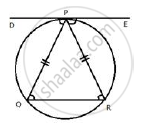Share

# Tangent at P to the Circumcircle of Triangle Pqr is Drawn. If the Tangent is Parallel to Side, Qr Show that δPqr is Isosceles. - Mathematics

Course

#### Question

Tangent at P to the circumcircle of triangle PQR is drawn. If the tangent is parallel to side, QR show that ΔPQR is isosceles.

#### SolutionDE is the tangent to the circle at P.
DE ∥ QR (Given)

∠EPR  = ∠PRQ (Alternate angles are equal)
∠DPQ = ∠PQR (Alternate angles are equal) ….. (i)
Let ∠DPQ = x and ∠EPR = y
Since the angle between a tangent and a chord through the point of contact is equal to the angle in the alternate segment

∴ ∠DPQ = ∠PRQ ……….. (ii) (DE is tangent and PQ is chord)
from (i) and (ii)
∠ PQR  = ∠PRQ
⇒ PQ = PR
Hence, triangle PQR is an isosceles triangle.

Is there an error in this question or solution?